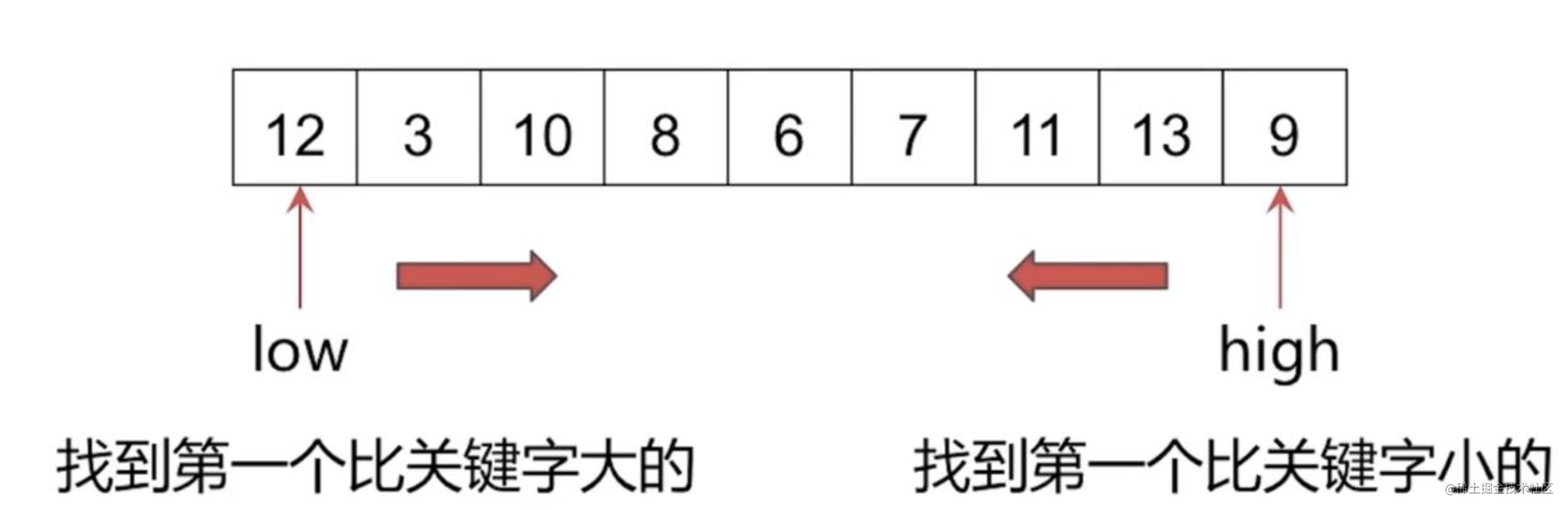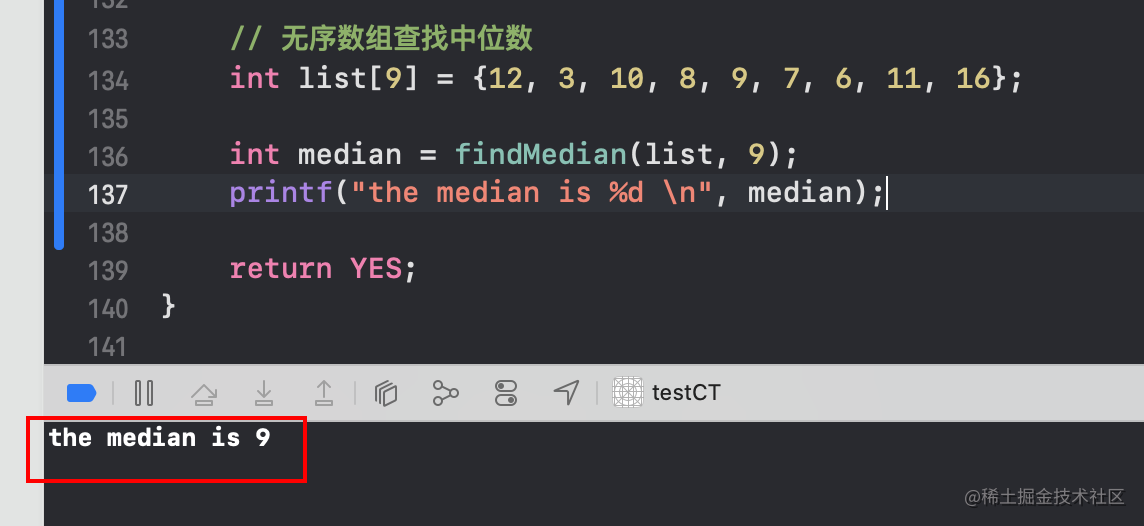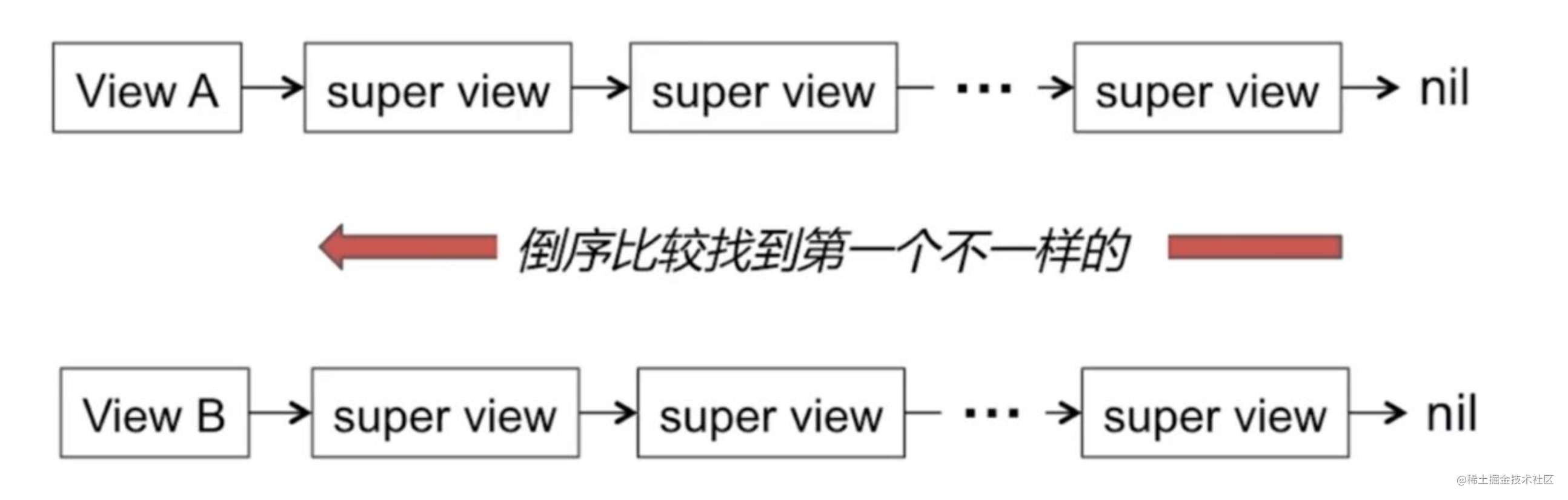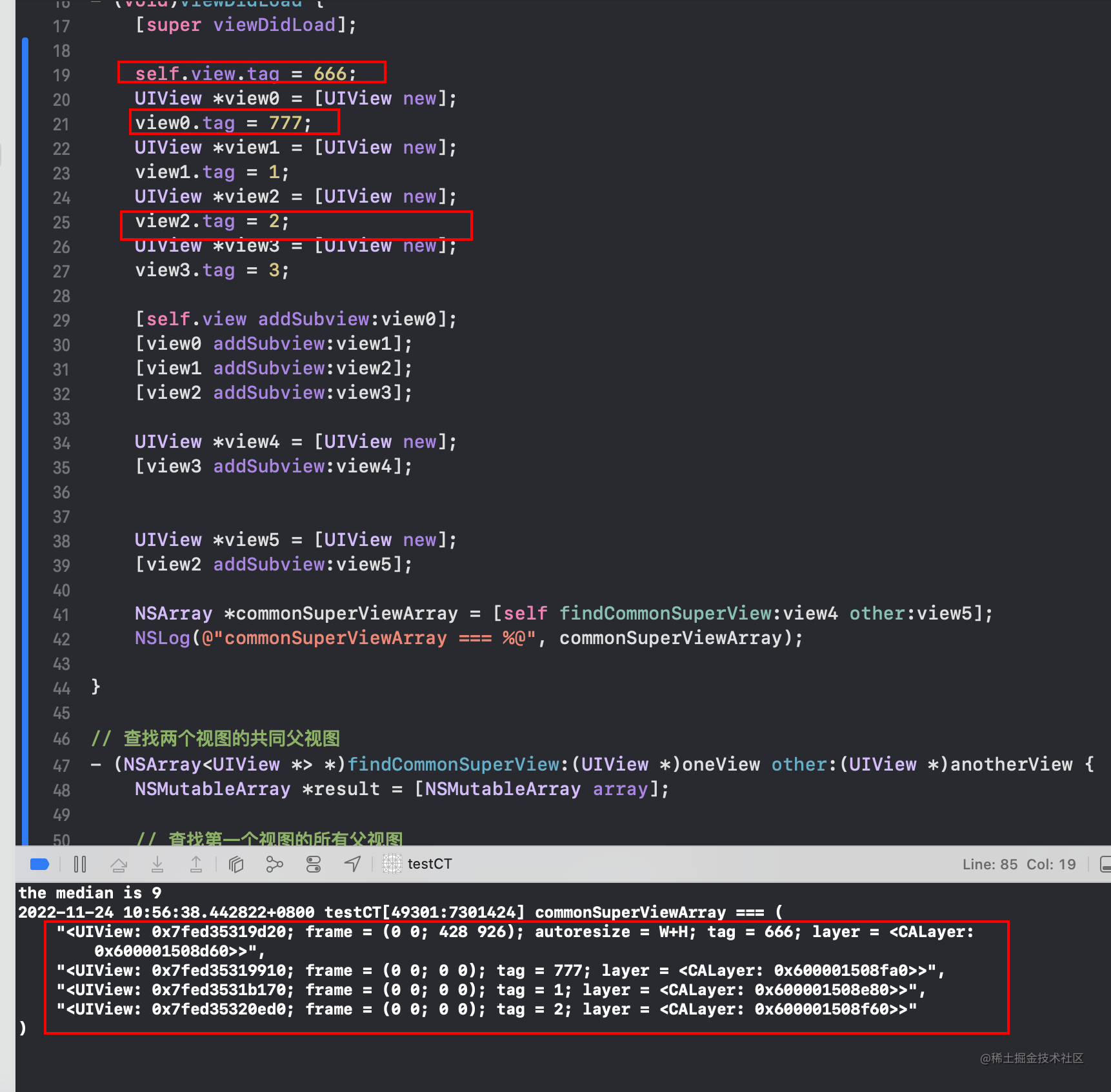# iOS老司机整理, iOSer必会的经典算法_2

## 前言

• iOS日常开发中, 算法使用的多吗? 实事求是的来说, 是不多的.
• 那算法的学习对iOSer来说, 就不需要了吗? 答案是很需要.
• iOS的日常开发中, 用到的算法确实不多, 但是算法在iOS开发里面的应用都被封装在各种常用API的内部了.
• 对算法的学习, 可以提高对iOS底层的理解与认识, 这些算法的知识是计算机技术领域通用的. 下面就iOS开发中一些经典的算法进行一个简单的梳理.
• 文章纯手打, 抛砖引玉, 如有错误还请评论区指正, 先行谢过了:)

# 1. 哈希算法, 在一个字符串中找到第一个, 只出现一次的字符.

• 在字符串 s 中找出第一个只出现一次的字符。如果没有，返回一个单空格。 s 只包含小写字母。 ``` 输入：s = "abaccdeff" 输出：'b'

``````char result = '\0';
// 1. 定义一个数组, 用来存储各个字母出现的次数
int array;

// 2. 对数组进行初始化操作
for (int i = 0; i < 256; i++) {
array[i] = 0;
}

// 3. 定义一个指针, 指向当前字符串头部
char *p = s;

// 4. 遍历每个字符, 在字母对应存储位置, 进行出现次数+1操作
while (*p != '\0') {
array[*(p++)]++;
}

// 5. 将P指针重新指向字符串头部
p = s;

// 6. 遍历每个字母出现次数, 遇到第一个出现次数为1的字符, 打印结果, 反之继续向后遍历
while (*p != '\0') {
if (array[*p] == 1) {
result = *p;
return result;
break;
}

p++;
}

return ' ';
``````

} ``` - 力扣链接

# 2. 求无序数组当中的中位数

## 2.1 排序算法 + 中位数

• 冒泡排序 快速排序 堆排序
• 中位数 ```当n为奇数时, (n + 1)/2 当n为偶数时, (n/2 + (n/2 + 1))/2```

## 2.2 利用快排思想(分治思想)

• 选取关键字, 高低指针交替扫描
• 找到第一个比关键字大的
• 找到第一个比关键字小的1. 任意挑一个元素, 以该元素为支点, 划分集合为两部分.
2. 如果左侧集合长度恰为`(n-1)/2`, 那么支点恰为中位数.
3. 如果`左侧长度<(n-1)/2`, 那么中位点在右侧; 反之, 中位数在左侧.
4. 进入相应的一侧继续寻找中位点.

5. 使用C语言的解答: ``` int findMedian(int a[], int aLen) { int low = 0; int high = aLen -1;

int mid = (aLen - 1) / 2; int div = PartSort(a, low, high);

while (div != mid) { if (mid < div) { // 左半区间找 div = PartSort(a, low, div - 1); } else { // 右半区间找 div = PartSort(a, div + 1, high); } }

// 找到了 return a[mid]; }

int PartSort(int a[], int start, int end) { int low = start; int high = end;

``````// 选取关键字
int key = a[end];

while (low < high) {
// 左边找比key大的值
while (low < high && a[low] <= key) {
++low;
}

// 右边找比key小的值
while (low < high && a[high] >= key) {
--high;
}

if (low < high) {
// 找到之后交换左右的值
int temp = a[low];
a[low] = a[high];
a[high] = temp;
}
}

int temp = a[high];
a[high] = a[end];
a[end] = temp;

return low;
``````

} ```

• 验证代码: ``` // 无序数组查找中位数 int list = {12, 3, 10, 8, 9, 7, 6, 11, 16};

int median = findMedian(list, 9); printf("the median is %d \n", median); ```# 3. 查找两个子视图的共同父视图

• 算法思路图解:• 使用Objective-C语言的解答: ```

self.view.tag = 666;     UIView view0 = [UIView new];     view0.tag = 777;     UIView view1 = [UIView new];     view1.tag = 1;     UIView view2 = [UIView new];     view2.tag = 2;     UIView view3 = [UIView new];     view3.tag = 3;

UIView *view4 = [UIView new];     [view3 addSubview:view4];

UIView *view5 = [UIView new];     [view2 addSubview:view5];

NSArray *commonSuperViewArray = [self findCommonSuperView:view4 other:view5];     NSLog(@"commonSuperViewArray === %@", commonSuperViewArray); }

// 查找两个视图的共同父视图 - (NSArray )findCommonSuperView:(UIView )oneView other:(UIView )anotherView {     NSMutableArray result = [NSMutableArray array];

// 查找第一个视图的所有父视图     NSArray arrayOne = [self findSuperViews:oneView];     // 查找第一个视图的所有父视图     NSArray arrayOther = [self findSuperViews:anotherView];

int i = 0;     // 越界限制条件     while (i < MIN(arrayOne.count, arrayOther.count)) {         // 倒序方式获取各个视图的父视图         UIView superOne = [arrayOne objectAtIndex:arrayOne.count - i - 1];         UIView superOhter = [arrayOther objectAtIndex:arrayOther.count - i - 1];

// 比较如果相等, 则为共同父视图         if (superOne == superOhter) {             [result addObject:superOne];             i++;         }         else {// 如果不相等, 则结束遍历             break;         }     }

return result; }

• (NSArray )findSuperViews:(UIView )view {     // 初始化为第一父视图     UIView *temp = view.superview;

// 保存结果的数组     NSMutableArray *result = [NSMutableArray array];

while (temp) {         [result addObject:temp];         // 顺着superview指针一直向上查找         temp = temp.superview;     }

return result; } ```#### 发文不易, 喜欢点赞的人更有好运气👍 :), 定期更新+关注不迷路~

ps：欢迎加入笔者18年建立的研究iOS审核及前沿技术的三千人扣群：662339934，坑位有限，备注“掘金网友”可被群管通过~